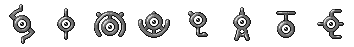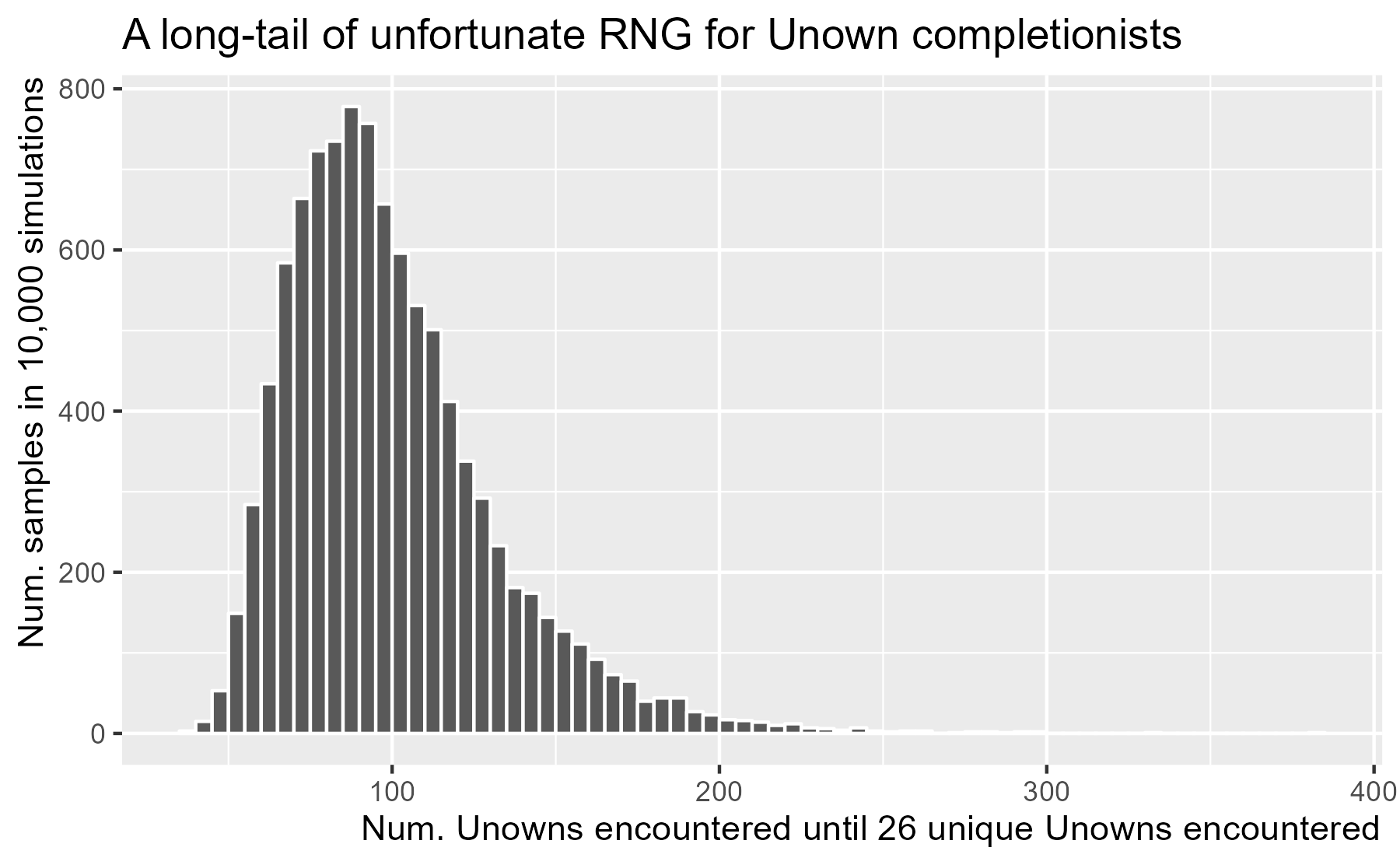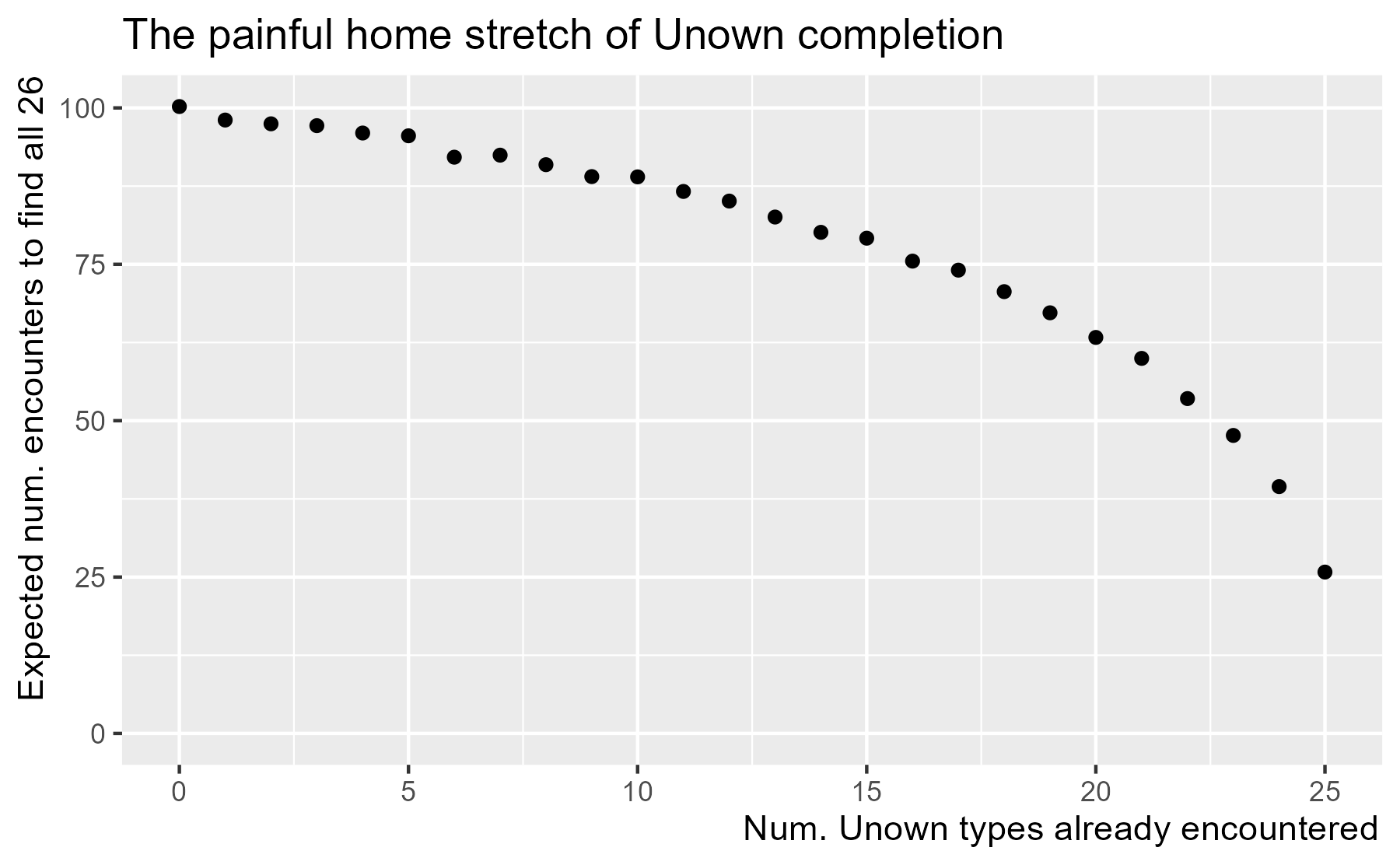# Simulating Unown encounter rates in Pokémon Go

Pokémon Go is an augmented reality game where people with smartphones walk around and catch Pokémon. As in the classic games, players are Pokémon “trainers” who have to travel around and collect creatures. Some types are rarer than others, some have regional habitats or biomes, and so players explore the game world to find and collect them. Pokémon Go “augments reality” having these Pokémon spawn in the game world as players travel around the real world. I like the game; I’ve reached level 30.On February 16, 2017, a second “generation” of Pokémon were released into the wild, adding dozens of new species to the game. The rarest among this new generation is Unown. As part of a cruel scheme to torture completionists, the incredibly rare Unown comes in 26 varieties—one for each letter of the alphabet. Which brings us to the statistical question behind this blog post: How long will it take to encounter all 26 types of Unowns (taking into account repeats)? Somewhat more formally, how many random encounters (i.e., draws while sampling with replacement) will it take until we have encountered all 26 Unowns?

## Unown collector’s problem

This general problem is called the coupon collector’s problem—hat tip to r/TheSilphRoad. The Wikipedia article for the problem includes a table which says the expected number of encounters for n = 26 is 101. (The analytic solution is actually 100.2, but we round up because there are no fractional encounters.) So problem solved?

Well, not quite. Suppose we had never heard of the coupon collector’s problem. Also, suppose that we want to get a sense of the uncertainty around the expected value. For example, how many encounters will it take for the unfortunate trainers in the 95th percentile? How might we tackle this problem?When analytic solutions are difficult or tedious, we can write simulations and get very good approximations. That’s what we’re going to do in R.

We will write a function to simulate a single exhaustive set of Unown encounters. The main workhorse is sample(..., replace = TRUE) to sample with replacement. Using R’s built-in LETTERS constant, we can simulate a batch of Unown encounters.

sample(LETTERS, size = 1, replace = TRUE)
#>  "B"
sample(LETTERS, size = 5, replace = TRUE)
#>  "X" "E" "C" "L" "E"
sample(LETTERS, size = 10, replace = TRUE)
#>   "B" "N" "Q" "M" "F" "V" "I" "J" "W" "D"


The question now is how many samples does it take to get the 26 different Unowns. An absolute, and frankly miraculous, lower bound on this number would be 26, so let’s draw 26 samples.

set.seed(151)  # For reproducible blogging
n_unique <- function(xs) length(unique(xs))

# Unowns in the batch
first_batch <- sample(LETTERS, size = 26, replace = TRUE)
first_batch
#>   "M" "Q" "D" "R" "B" "X" "H" "C" "Q" "E" "F" "K" "V" "S" "R" "P" "N" "C" "F"
#>  "E" "L" "A" "X" "J" "Q" "O"

# Number of unique ones
n_unique(first_batch)
#>  19

# Number of remaining Unowns we have not encountered yet
leftover <- 26 - n_unique(first_batch)
leftover
#>  7


We encountered 19 unique Unowns from the first batch of samples. The best-case lower bound for the number of the encounters remaining is now 7, so let’s take 7 more samples.

second_batch <- sample(LETTERS, size = leftover, replace = TRUE)
second_batch
#>  "N" "L" "I" "V" "L" "H" "L"

# Combine the batches
both_batches <- c(first_batch, second_batch)
n_unique(both_batches)
#>  20

leftover <- 26 - n_unique(both_batches)
leftover
#>  6


We found 1 new Unowns in this batch, and we have encountered 20 unique ones so far from 33 total samples. That means the lower bound is now 6.

third_batch <- sample(LETTERS, size = leftover, replace = TRUE)
third_batch
#>  "V" "J" "D" "D" "C" "D"

all_batches <- c(both_batches, third_batch)
n_unique(all_batches)
#>  20

leftover <- 26 - n_unique(all_batches)
leftover
#>  6


We found 0 new Unowns in this—

Actually, this is getting tedious. We all know where this process is going: Take a sample, see how many you have left to find, take another sample of that size, etc. until you have 0 left to find. Pretty simple? Great! Now, I don’t have to explain how the while loop inside the function works.

simulate_unown <- function() {
# Use a sequence of numbers instead of LETTERS
n_unowns <- 26
unown_set <- seq_len(n_unowns)

n_unique <- 0
encountered <- character(0)

# Take 26 samples on first iteration, 26 - n_unique on next iteration, etc.
while (n_unowns - n_unique > 0) {
batch <- sample(unown_set, size = n_unowns - n_unique, replace = TRUE)
encountered <- c(encountered, batch)
n_unique <- length(unique(encountered))
}

length(encountered)
}


Each call of the function simulates a process of encountering all 26 Unowns, returning how many encounters were required to find them all.

simulate_unown()
#>  59
simulate_unown()
#>  108
simulate_unown()
#>  103


We use replicate() to call this function thousands of times.

simulation <- replicate(10000, simulate_unown())


We can get summary statistics and other quantiles for these simulations.

summary(simulation)
#>    Min. 1st Qu.  Median    Mean 3rd Qu.    Max.
#>    39.0    78.0    94.0   100.4   116.0   382.0
quantile(simulation, probs = c(.05, .10, .25, .50, .75, .90, .95))
#>     5%    10%    25%    50%    75%    90%    95%
#>  60.00  66.00  78.00  94.00 116.00 142.10 161.05


The mean in our simulations 100.4 is very close to the analytic solution of 100.2. The median 94 is less than the mean, which is a bit of good news: More than half of players will hit 26 in less than the expected 100 encounters. The bad news is that there is a long tail to these simulations, as the RNG gods have cursed one player by requiring 382 encounters.

We can visualize the distribution with a histogram.

library(ggplot2)
p1 <- ggplot(data.frame(x = simulation)) +
aes(x = x) +
geom_histogram(binwidth = 5, color = "white", center = 102.5) +
labs(x = "Num. Unowns encountered until 26 unique Unowns encountered",
y = "Num. samples in 10,000 simulations") +
theme(axis.title.x = element_text(hjust = .995),
axis.title.y = element_text(hjust = .995)) +
ggtitle("A long-tail of unfortunate RNG for Unown completionists")
p1I haven’t seen any of these Pokémon in the month since they were added to the game. Assuming I see an Unown every month, I can expect to see them all in 100 encounters over the course of 8.3 years. As I said, a cruel joke for completionists.

## What? SIMULATE_UNOWN is evolving!

One nice thing about using simulations to compute statistics is that we can easily modify the simulation function to answer related questions. For example:

• The above simulation assumed that we can catch the Unowns 100% of the time. What if we fail on 5% of the encounters?
• Two more Unowns were added to the series in the third Pokémon generation. What is the expected number of encounters for 28 Unowns?
• Suppose we already have 20 Unowns. How many more encounters are required to collect the remaining 6?

We can add some parameters to our function to address these questions. To simulate catching, we sample a TRUE or FALSE value for each Unown sampled. The prob argument for sample() lets us assign probabilities to elements in the sample, so we can use c(p_catch, 1 - p_catch) as probabilities for sampling TRUE or FALSE—that is, catching a Pokémon.

To handle already-captured Unowns, we add this information to the initial values for encountered and n_unique to give those values a head start before the encounter/catch loop. Afterwards, we have to adjust our encounter count by that head start.

# Defaults to 26 unowns, 0 already caught, and 100% success rate
simulate_unown_catches <- function(n_unowns = 26, n_already = 0, p_catch = 1) {
unown_set <- seq_len(n_unowns)
catch_probs <- c(p_catch, 1 - p_catch)

# Take into account any previously caught ones

# Encounter/catch loop
while (n_unowns - n_unique > 0) {
batch <- sample(unown_set, size = n_unowns - n_unique, replace = TRUE)

# Simulate catching success for each Unown
catches <- sample(c(TRUE, FALSE), size = n_unowns - n_unique,
replace = TRUE, prob = catch_probs)
caught_in_batch <- batch[catches]

encountered <- c(encountered, batch)
caught <- c(caught, caught_in_batch)
n_unique <- length(unique(caught))
}

}


With the default settings, the function should reproduce the original behavior and give us similar results.

simulation2 <- replicate(10000, simulate_unown_catches())
summary(simulation2)
#>    Min. 1st Qu.  Median    Mean 3rd Qu.    Max.
#>    34.0    78.0    95.0   100.3   116.0   330.0


We should expect the average number of required encounters to catch all 26 Unowns to increase by 1.05 if there’s a 95% catch rate. Our simulations confirm this intuition.

simulation_95_rate <- replicate(
n = 10000,
expr = simulate_unown_catches(n_unowns = 26, p_catch = .95)
)

summary(simulation_95_rate)
#>    Min. 1st Qu.  Median    Mean 3rd Qu.    Max.
#>    38.0    82.0   100.0   105.9   123.0   329.0


We can also simulate the case for 28 Unowns with a 100% encounter rate, and see that the average number of required encounters increases by approximately 5.

simulation_28_unowns <- replicate(
n = 10000,
expr = simulate_unown_catches(n_unowns = 28, p_catch = 1)
)

summary(simulation_28_unowns)
#>    Min. 1st Qu.  Median    Mean 3rd Qu.    Max.
#>    42.0    86.0   104.0   110.4   128.0   451.0


Finally, we can simulate the home stretch of Unown completion where we have 20 Unowns with 6 more to go.

simulation_last_6 <- replicate(
n = 10000,
expr = simulate_unown_catches(n_unowns = 26, n_already = 20, p_catch = 1)
)

summary(simulation_last_6)
#>    Min. 1st Qu.  Median    Mean 3rd Qu.    Max.
#>   10.00   42.00   58.00   64.01   79.00  290.00


This result is interesting: It says that we will spend the majority of our time working on the last 6 Unowns.

## Simulate ‘em all

A natural next step is to run many simulations over a range of parameter values. Here, we can study the home-stretch behavior by running a simulation for each value of n_already from 0 to 25.

We will create a function to simulate 2000 home-stretch samples of required encounters for a given number of starting Unowns. This function will return a dataframe with one row per simulation sample. We use Map() to apply this function for each value of n_already from 0 to 25.

library(dplyr, warn.conflicts = FALSE)

n_sims <- 2000
sim_results <- replicate(
n = n_sims,
expr = simulate_unown_catches(n_unowns = 26, n_already, p_catch = 1)
)

# Package everything together in a dataframe
tibble::tibble(
simulation = seq_len(n_sims),
n_encounters = sim_results
)
}

results <- Map(simulate_2k, n_already = 0:25)
df_results <- bind_rows(results)
df_results
#> # A tibble: 52,000 × 3
#>        <int>      <int>        <int>
#>  1         0          1          112
#>  2         0          2           69
#>  3         0          3          128
#>  4         0          4           67
#>  5         0          5          108
#>  6         0          6           67
#>  7         0          7          115
#>  8         0          8          124
#>  9         0          9           66
#> 10         0         10           88
#> # … with 51,990 more rows


We can now plot the expected number of encounters for each starting value. Variance from random simulation keeps the points from following a neat curve, so let’s just appreciate the main trends in the results.

ggplot(df_results) +
aes(x = n_already, y = n_encounters) +
stat_summary(fun = mean, geom = "point") +
labs(
x = "Num. Unown types already encountered",
y = "Expected num. encounters to find all 26"
) +
expand_limits(y = 0) +
theme(
axis.title.x = element_text(hjust = .995),
axis.title.y = element_text(hjust = .995)
) +
ggtitle("The painful home stretch of Unown completion")Because the analytic solution for finding 26 Unowns starting from 0 is 100, we can also read the y-axis as a percentage. In other words, 60% of the work (number of encounters out of 100) will be spent on the last 5 Unowns.

## Simulation as a way to learn statistics

An underlying theme for this post is best summarized by a line from a talk called Statistics for Hackers:

“If you can write for loops, you can do statistics.” — Statistics for Hackers

Simulation provides a way for “hackers” to leverage one skill (programming) to learn another domain (statistics). I myself find that I have trouble learning statistics from equations alone. I need code to play with a problem and develop intuitions about it.

All of these points about the nice features of simulation must be painfully obvious to professional statisticians. But strangely, I only used simulations once or twice in my statistics courses, so it wasn’t part of my statistical tool kit. Indeed, I had the usefulness of simulation impressed upon me last year by Gelman and Hill’s textbook. The book advises the reader to not worry about analytically computing statistics for tricky calculations—for example, trying to estimate a 95% prediction interval for the income difference between two groups when the underlying statistical model used log-dollars. Just have the model generate thousands for predictions, run the transformation, and find the interval on the transformed data. The book also uses simulation as a sneaky backdoor into informal Bayesian statistics: Measure uncertainty by simulating new data from perturbed model parameters. This idea made enough sense to me to lure me into the chapters on formal Bayesian inference and learn that statistical framework.

Last knitted on 2022-05-27. Source code on GitHub.1

1. .session_info
#> ─ Session info ───────────────────────────────────────────────────────────────
#>  setting  value
#>  version  R version 4.2.0 (2022-04-22 ucrt)
#>  os       Windows 10 x64 (build 22000)
#>  system   x86_64, mingw32
#>  ui       RTerm
#>  language (EN)
#>  collate  English_United States.utf8
#>  ctype    English_United States.utf8
#>  tz       America/Chicago
#>  date     2022-05-27
#>  pandoc   NA
#>
#> ─ Packages ───────────────────────────────────────────────────────────────────
#>  package     * version date (UTC) lib source
#>  assertthat    0.2.1   2019-03-21  CRAN (R 4.2.0)
#>  cli           3.3.0   2022-04-25  CRAN (R 4.2.0)
#>  colorspace    2.0-3   2022-02-21  CRAN (R 4.2.0)
#>  crayon        1.5.1   2022-03-26  CRAN (R 4.2.0)
#>  DBI           1.1.2   2021-12-20  CRAN (R 4.2.0)
#>  digest        0.6.29  2021-12-01  CRAN (R 4.2.0)
#>  dplyr       * 1.0.9   2022-04-28  CRAN (R 4.2.0)
#>  ellipsis      0.3.2   2021-04-29  CRAN (R 4.2.0)
#>  evaluate      0.15    2022-02-18  CRAN (R 4.2.0)
#>  fansi         1.0.3   2022-03-24  CRAN (R 4.2.0)
#>  farver        2.1.0   2021-02-28  CRAN (R 4.2.0)
#>  generics      0.1.2   2022-01-31  CRAN (R 4.2.0)
#>  ggplot2     * 3.3.6   2022-05-03  CRAN (R 4.2.0)
#>  git2r         0.30.1  2022-03-16  CRAN (R 4.2.0)
#>  glue          1.6.2   2022-02-24  CRAN (R 4.2.0)
#>  gtable        0.3.0   2019-03-25  CRAN (R 4.2.0)
#>  here          1.0.1   2020-12-13  CRAN (R 4.2.0)
#>  highr         0.9     2021-04-16  CRAN (R 4.2.0)
#>  knitr       * 1.39    2022-04-26  CRAN (R 4.2.0)
#>  labeling      0.4.2   2020-10-20  CRAN (R 4.2.0)
#>  lifecycle     1.0.1   2021-09-24  CRAN (R 4.2.0)
#>  magrittr      2.0.3   2022-03-30  CRAN (R 4.2.0)
#>  munsell       0.5.0   2018-06-12  CRAN (R 4.2.0)
#>  pillar        1.7.0   2022-02-01  CRAN (R 4.2.0)
#>  pkgconfig     2.0.3   2019-09-22  CRAN (R 4.2.0)
#>  purrr         0.3.4   2020-04-17  CRAN (R 4.2.0)
#>  R6            2.5.1   2021-08-19  CRAN (R 4.2.0)
#>  ragg          1.2.2   2022-02-21  CRAN (R 4.2.0)
#>  rlang         1.0.2   2022-03-04  CRAN (R 4.2.0)
#>  rprojroot     2.0.3   2022-04-02  CRAN (R 4.2.0)
#>  rstudioapi    0.13    2020-11-12  CRAN (R 4.2.0)
#>  scales        1.2.0   2022-04-13  CRAN (R 4.2.0)
#>  sessioninfo   1.2.2   2021-12-06  CRAN (R 4.2.0)
#>  stringi       1.7.6   2021-11-29  CRAN (R 4.2.0)
#>  stringr       1.4.0   2019-02-10  CRAN (R 4.2.0)
#>  systemfonts   1.0.4   2022-02-11  CRAN (R 4.2.0)
#>  textshaping   0.3.6   2021-10-13  CRAN (R 4.2.0)
#>  tibble        3.1.7   2022-05-03  CRAN (R 4.2.0)
#>  tidyselect    1.1.2   2022-02-21  CRAN (R 4.2.0)
#>  utf8          1.2.2   2021-07-24  CRAN (R 4.2.0)
#>  vctrs         0.4.1   2022-04-13  CRAN (R 4.2.0)
#>  withr         2.5.0   2022-03-03  CRAN (R 4.2.0)
#>  xfun          0.31    2022-05-10  CRAN (R 4.2.0)
#>
#>   C:/Users/Tristan/AppData/Local/R/win-library/4.2
#>   C:/Program Files/R/R-4.2.0/library
#>
#> ──────────────────────────────────────────────────────────────────────────────


Tags:

Posted: